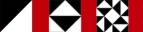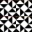Next Higher-Order Parsing 6

# 例：グラフを描いてみるExample: graphing program

• ユーザがウェブから式を入力する

Suppose we want to read a web user's input

• こんな感じの数式を読んで

It will be a mathematical function, like

```        (x^2 + 3*x)* sin(x * 2) + 14
```
• その数式のグラフを出力したい

We will emit a web page with a graph of their function

• 手軽なやり方

Easy solution:

• evalを使って入力をPerlコードにコンパイルする

Use eval to turn user input into compiled Perl code

 NextCopyright © 2007 M. J. Dominus